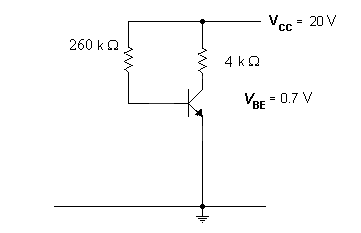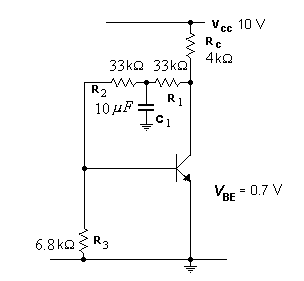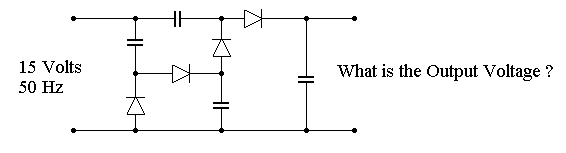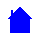EL10C TUTORIAL #1   Saturday, 14 June 2003

1. The current gain for the transistor ranges from 40 to 100. Calculate the variation in Vce that is possible for the circuit2. The same transistor is used in this circuit. Calculate the variations in collector current and emitter and collector voltages that are possible.3. Again we are using the same transistor. Calculate the variations in collector current and collector voltage that are possible4. Calculate the voltage on the base and emitter of the transistor for the circuit below5. Find the output voltage for the circuit below.EL10C Homepage